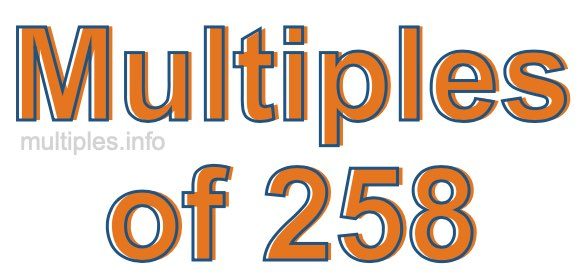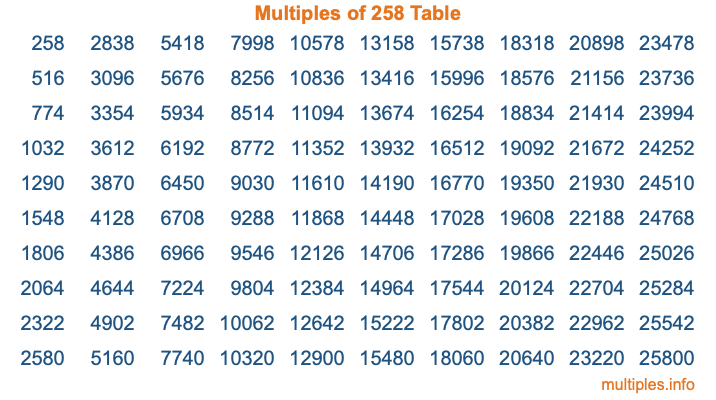Multiples of 258Welcome to the Multiples of 258 page. Here we will first teach you everything you will ever need to know about the multiples of 258, and then give you a study guide summary of everything we taught you to make sure you remember it all. Use this page to look up facts and learn information about the multiples of 258. This page will make you a multiples of two hundred fifty-eight expert!

Definition of Multiples of 258
Multiples of 258 are all the numbers that when divided by 258 equal an integer. Each of the multiples of 258 are called a multiple. A multiple of 258 is created by multiplying 258 by an integer.

Therefore, to create a list of multiples of 258, you start with 1 multiplied by 258, then 2 multiplied by 258, then 3 multiplied by 258, and so on for as long as you want. Thus, the list of the first five multiples of 258 is 258, 516, 774, 1032, and 1290. To see a larger list of multiples of 258, see the printable image of Multiples of 258 further down on this page. We also have a category where you can choose any nth multiple of 258.

Multiples of 258 Checker
The Multiples of 258 Checker below checks to see if any number of your choice is a multiple of 258. In other words, it checks to see if there is any number (integer) that when multiplied by 258 will equal your number. To do that, we divide your number by 258. If the the quotient is an integer, then your number is a multiple of 258.

Is  a multiple of 258?

Least Common Multiple of 258 and ...
A Least Common Multiple (LCM) is the lowest multiple that two or more numbers have in common. This is also called the smallest common multiple or lowest common multiple and is useful to know when you are adding our subtracting fractions. Enter one or more numbers below (258 is already entered) to find the LCM.

Check out our LCM Calculator if you need more details about the Least Common Multiple or if you need the LCM for different numbers for adding and subtraction fractions.

nth Multiple of 258
As we stated above, 258 is the first multiple of 258, 516 is the second multiple of 258, 774 is the third multiple of 258, and so on. Enter a number below to find the nth multiple of 258.

th multiple of 258

Multiples of 258 vs Factors of 258
258 is a multiple of 258 and a factor of 258, but that is where the similarities end. All postive multiples of 258 are 258 or greater than 258. All positive factors of 258 are 258 or less than 258.

Below is the beginning list of multiples of 258 and the factors of 258 so you can compare:

Multiples of 258: 258, 516, 774, 1032, 1290, etc.

Factors of 258: 1, 2, 3, 6, 43, 86, 129, 258

As you can see, the multiples of 258 are all the numbers that you can divide by 258 to get a whole number. The factors of 258, on the other hand, are all the whole numbers that you can multiply by another whole number to get 258.

It's also interesting to note that if a number (x) is a factor of 258, then 258 will also be a multiple of that number (x).

Multiples of 258 vs Divisors of 258
The divisors of 258 are all the integers that 258 can be divided by evenly. Below is a list of the divisors of 258.

Divisors of 258: 1, 2, 3, 6, 43, 86, 129, 258

The interesting thing to note here is that if you take any multiple of 258 and divide it by a divisor of 258, you will see that the quotient is an integer.

Multiples of 258 Table
Below is an image of the first 100 multiples of 258 in a table. The table is in chronological order, column by column. The first column has the first ten multiples of 258, the second column has the next ten multiples of 258, and so on.The Multiples of 258 Table is also referred to as the 258 Times Table or Times Table of 258. You are welcome to print out our table for your studies.

Negative Multiples of 258
Although not often discussed or needed in math, it is worth mentioning that you can make a list of negative multiples of 258 by multiplying 258 by -1, then by -2, then by -3, and so on, to get the following list of negative multiples of 258:

-258, -516, -774, -1032, -1290, etc.

Multiples of 258 Summary
Below is a summary of important Multiples of 258 facts that we have discussed on this page. To retain the knowledge on this page, we recommend that you read through the summary and explain to yourself or a study partner why they hold true.

There are an infinite number of multiples of 258.

A multiple of 258 divided by 258 will equal a whole number.

258 divided by a factor of 258 equals a divisor of 258.

The nth multiple of 258 is n times 258.

The largest factor of 258 is equal to the first positive multiple of 258.

258 is a multiple of every factor of 258.

258 is a multiple of 258.

A multiple of 258 divided by a divisor of 258 equals an integer.

258 divided by a divisor of 258 equals a factor of 258.

Any integer times 258 will equal a multiple of 258.

Multiples of a Number
Here you can get the multiples of another number, all with the same attention to detail as we did for multiples of 258 on this page.

Multiples of
Multiples of 259
Did you find our page about multiples of two hundred fifty-eight educational? Do you want more knowledge? Check out the multiples of the next number on our list!